Select Page

# CBSE 12 Science Solutions for MCQ Maths Differential Equations in English

CBSE 12 Science Solutions for MCQ Maths Differential Equations in English to enable students to get Solutions in a narrative video format for the specific question.

Expert Teacher provides CBSE 12 Science Solutions for MCQ Maths Differential Equations through Video Solutions in English language. This video solution will be useful for students to understand how to write an answer in exam in order to score more marks. This teacher uses a narrative style for a question from Differential Equations not only to explain the proper method of answering question, but deriving right answer too.

Please find the question below and view the Solution in a narrative video format.

Question:

Solution Video in English:

You can select video Solutions from other languages also. Please check Solutions in ( Hindi )

## Similar Questions from CBSE, 12th Science, Maths, Differential Equations

Question 1 : Find the particular solution of the differential equation :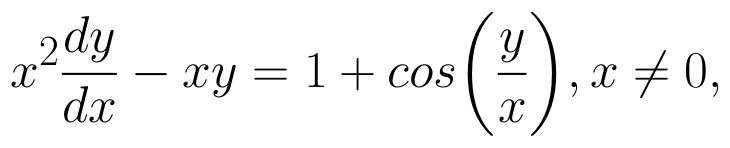when x = 1,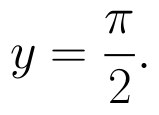(View Answer Video)

Question 2 : Find the general solution of the following differential equation :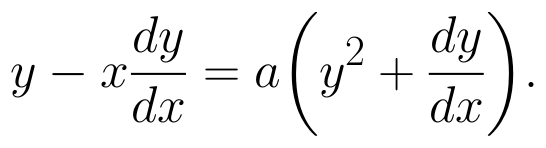(View Answer Video)

Question 3 : Solve the differential equation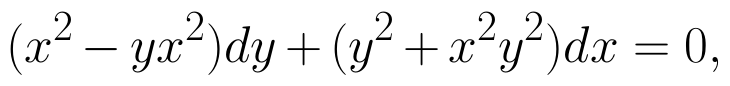given that y = 1 when x = 1. (View Answer Video)

Question 4 : Form the differential equation of equation y = a cos 2x + b sin 2x, where a and b are constant. (View Answer Video)

Question 5 : Write the sum of the order and degree of the differential equation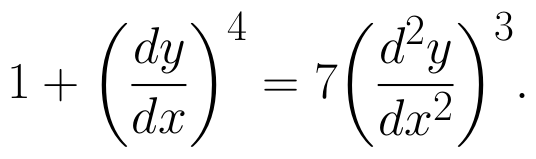(View Answer Video)

### Three Dimensional Geometry

Question 1 : Find the direction cosines of the line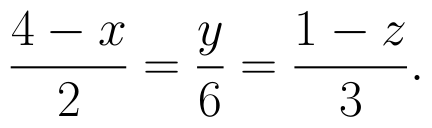(View Answer Video)

Question 2 : Find the vector equation of a plane which is at a distance of 5 units from the origin and its normal vector is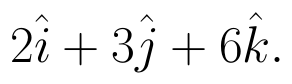(View Answer Video)

Question 3 : Find the distance of point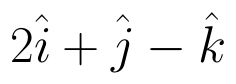from the  plane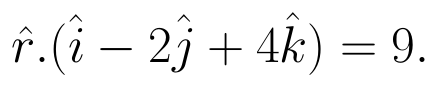(View Answer Video)

Question 4 : Find the vector and cartesian forms of the equation of the plane passing through the point (1, 2, -4) and parallel to the lines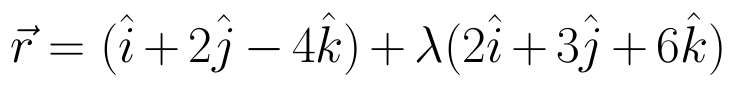and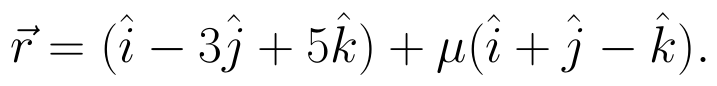Also, find the distance of the point (9, -8, -10) from the plane thus obtained. (View Answer Video)

Question 5 : Find the equation of the plane with intercept 3 on the y-axis and parallel to ZOX plane. (View Answer Video)

### Linear Programming

Question 1 : The objective function is maximum or minimum, which lies on the boundary of the feasible region. (View Answer Video)

### Integrals

Question 1 : Find :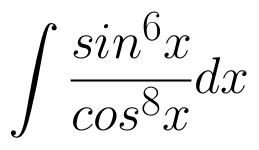(View Answer Video)

Question 2 : Evaluate :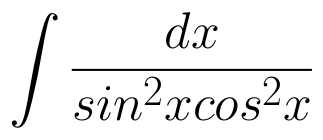(View Answer Video)

Question 3 : Evaluate :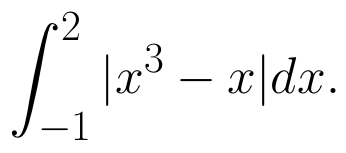(View Answer Video)

Question 4 : Evaluate :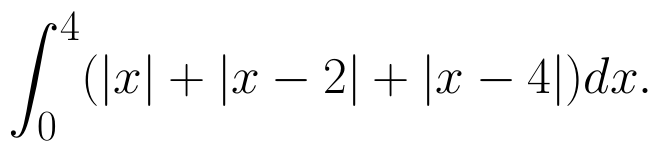(View Answer Video)

Question 5 : Find the integral of the function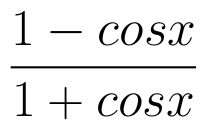. (View Answer Video)